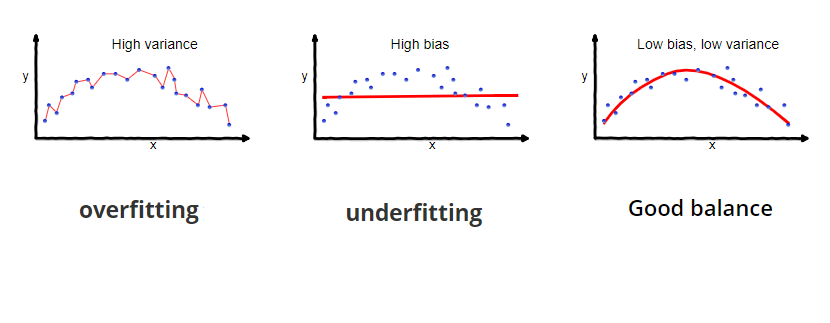Hey - Nick here! This page is a free excerpt from my new eBook Pragmatic Machine Learning, which teaches you real-world machine learning techniques by guiding you through 9 projects.

In this tutorial, you will learn about the bias-variance tradeoff in machine learning, which is a core concept that will allow you to understand whether your model is properly fitted to its training data.

## What is the Bias-Variance Tradeoff?

The bias-variance tradeoff refers to the point where you add too much model sophistication by hyper-tuning it to be accurate on your training data. When this happens, your model does not generalize well to other data sets (namely, the test data).

You can tell when a model has passed the bias-variance tradeoff when the predictions it makes on the training data are significantly better than its predictions on the test data. This phenomenon is known as `overfitting`.

Visualizations can be very helpful in understanding the bias-variance tradeoff. Consider the following diagram:As you can see, the model on the left has been overfitted to the training data. The model in the middle has been underfitted. Neither of these models would be very useful in making predictions using an outside data set.

The model on the right, however, has a nice balance of low bias and low variance, which means that it is well-suited for predictions on outside data sets.

## Final Thoughts

In this tutorial, you learned about the bias-variance tradeoff, which is a core concept that needs to be understood by machine learning practitioners.

Here is a brief summary of what we discussed in this tutorial:

• What the bias-variance tradeoff means
• How overfitting (high variance) and underfitting (high bias) can cause problems when attempting to make predictions on outside data sets